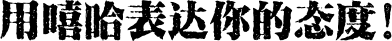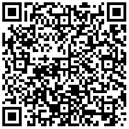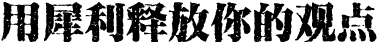用嘻哈表达你的态度2017新媒体人气榜点评有礼 助力你的公益2017新媒体口碑榜×
×
×
×
×
×
×
×
×
×
×
×
×
×
×
×
×
×
×
×
×
×
×
×
×
×
×
×
×
×
×
×
×
×
×
×
×
×
×